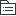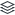# 第四章 美术基础理论

23
4

23
|
4

## 相关模板推荐

•## 美术基础理论 —— 作品大纲

•美术概论
• 美术的基本特征
• 造型性
• 运用一定的物质材料，塑造出欣赏者可以通过观察直接感受到的艺术形象
• 静态性
• 所有美术作品，都是以静态形式呈现的
• 表现性
• 表现形象的内在意蕴，是重要的审美特征
• 视觉性
• 审美主体与对象之间的感知媒介主要是视觉
• 美术门类的划分
• 绘画
• 建筑
• 雕塑
• 工艺美术
• 园林
• 书法
• 篆刻
•素描
• 素描的概念
• 素描是一切绘画的基础
• 素描的分类
• 表现手法
• 光影素描
• 结构素描
• 白描
• 速写
• 目的和功能
• 创作素描
• 习作素描
• 表现内容
• 静物
• 动物
• 风景
• 人像
• 及人体素描
• 素描绘画的基本因素
• 结构
• 结构是指决定物象外在形态的内部结构，各个部位之间的组合构成关系以及构成基本形的几何结构。
• 形体
• 形体是指物体的形状和体积，是体现物体高、宽、深立体性质的造型因素。
• 比例
• 比例是指物体的形体结构的尺度关系
• 明暗
• 明暗是表现形体结构空间透视、光影变化的一种造型手段
• 三面
• 亮面、灰面、暗面
• 五调
• 亮色调
• 包括高光
• 灰色调
• 中间色面
• 明暗交界线
• 暗色调
• 包括反光
• 投影
• 空间
• 素描能力培养遵循的基本原则
• 训练原则
• 目标明确、循序渐进
• 反复巩固、有的放矢
• 结合自己水平定出计划
• 由浅到深，不可急于求成
• 观察与表现原则
• 从整体出发
• 到局部
• 再回到整体
• 素描的表现方法
• 用线条
• 结构素描
• 用光影
• 明暗素描
• 用线和面结合
• 素描的绘画步骤
• 经营构图，确定轮廓
• 深入刻画，塑造形象
• 调整统一，完美画面
•速写
• 速写的定义
• 一种快速的写生方法。
• 速写的分类
• 速写的主要表现形式
• 以线条为主的速写
• 明暗速写
• 线面结合的速写
• 人物速写的基本步骤
• 观察
• 构图
• 局部刻画
•透视与解剖
• 透视
• 透视的基本原理
• 视平线
• 是人假定出来的一条直线，就是与画者眼睛平行的水平线
• 心点
• 画着眼睛正对视平线的一点，有时心点与消失点是重合的
• 视点
• 是作画者眼睛的位置，也是视线观察的出发点。
• 视中线
• 就是视点和心点相连，与视平线成直角的线。
• 消失点
• 物体在视平线上随着观察距离而产生的渐变消失的最小状态。
• 透视现象
• 平行透视
• 各管物象的前面与画面平行
• 成角透视
• 客观物象的任何一个面都不与画面平行，但底面与地平面平行，视线上有两个消失点的透视现象。
• 斜角透视
• 次种透视的形成是因为静物没有任何一条边缘或面块与画面平行，相对于画面，静物是倾斜的。
• 构图
• 构图的基本原则
• 均衡与对称
• 变化与统一
• 构图的方法
• 画面主体图形的位置
• 非主题图形的位置及其与主题图形的关系
• 画面底形的位置及其与图形的关系
• 构图的原则
• 美术作品中的一切，都应当从属于作品基本内容的表达
• 美术作品中的一切形式因素，都应当相互保持联系
• 作品应当通过构图，形成一个吸引观众最大注意力的视觉中心
• 一个画面的构图结构是既有变化又有统一的整体，应利用形体、明暗、线条、色彩等因素的对比协调规律及空间处理、体积结合、节奏韵律等手段，使作品形式新颖生动，并由最强的艺术表现力
• 安排画面物体，处理细节，应当避免一切偶然性，注意与主题表现得密切联系
• 应用构图中得均衡，以表现稳定和静止，应用构图中得不均衡，以表现不稳定和运动
• 作品得尺寸得大小，应配合它得内容；画幅得长宽比例应服从构图得需要
• 构图的基本形式
• 均衡式
• S形构图
• 对称式
• 金字塔式构图
• 解剖
• 人体共有206块骨骼
• 颅骨
• 脑颅
• 面颅
• 躯干骨
• 脊柱
• 胸廓
• 盆骨
• 四肢骨
• 上肢
• 肩部
• 上臂
• 前臂和手
• 下肢
• 大腿
• 小腿和足
• 人体比例
• 三庭五眼
• 站七坐五蹲三半
•色彩
• 色彩的分类
• 三原色
• 红黄蓝
• 间色
• 橙绿紫
• 复色
• 再间色、三次色
• 色彩三要素
• 色相
• 色泽 色彩的相貌
• 明度
• 明暗程度
• 纯度
• 饱和程度
• 色彩现象与规律
• 光源色
• 固有色
• 环境色
• 中性色
• 黑、白
• 同类色
• 同类色对比是明度和纯度对比
• 临近色
• 色彩的对比
• 色相对比
• 明度对比
• 纯度对比
• 色彩的鲜艳与浑浊程度对比
• 补色对比
• 红对绿
• 冷暖对比
• 面积对比
•图案
• 图案的概念
• 图案是实用和装饰相结合的一种美术形式
• 图案的特征
• 三要素
• 造型
• 色彩
• 构成
• 图案设计“六要”
• 内容与形式要相适应
• 局部与整体要相适应
• 图案与使用的环境要相适应
• 图案与生活环境要相适应
• 物质材料与用途要相适应
• 图案与生产条件的制约要相适应
• 图案的分类
• 按所占空间
• 平面图案
• 立体图案
• 按历史
• 原始社会图案
• 传统图案
• 现代图案
• 社会关系
• 宫廷工艺美术图案
• 民间工艺美术图案
• 图案形式美的原理法则
• 变化与统一
• 条理与反复
• 对称与平衡
• 对比与调和
• 节奏与韵律
• 图案的构成与组织
• 单独纹样
• 对称式纹样
• 绝对对称
• 相对对称
• 均衡式纹样
• 适合纹样
• 填充纹样
• 角隅（yu）纹样
• 边饰纹样
• 连续纹样
• 二方连续
• 上下或左右
• 四方连续
• 上下左右
•美术字
• 美术字的含义
• 美术字是经过加工、美化、装饰而成的文字
• 装潢艺术四要素
• 构图
• 图画
• 美术字
• 色彩
• 美术字分类
• 字体上来分
• 宋体
• 黑体
• 浑厚稳健，所有笔画粗细相等，方头端尾，转角处不留钝角
• 仿宋体
• 变体美术字
• 报头美术字
• 商业美术字
• 美术字的审美原则
• 思想性
• 可读性
• 艺术性
• 美术字的作用
• 美术字是社会政治、经济发展所必需的宣传工具之一。
• 美术字的艺术感染力具有美化人民生活的作用。
• 美术字的写法
• 打格
• 布局
• 定骨架
• 双钩字形
• 填色
•简笔画
• 简笔画的概念
• 简笔画是一种利用简单的点、线、形等绘画要素来表现物象基本特征的绘画形式。
• 用简单大方的笔法，提取客观形象最典型、最突出的主要特点，表现出既有概括性又有可识性和示意性的绘画。
• 简笔画的构成要素
• 线
• 简笔画的造型方法
• 观察分析物象
• 用基本形概括物象
• 运用夸张的手法